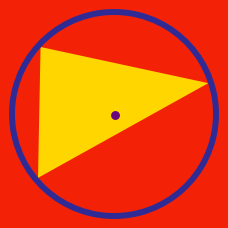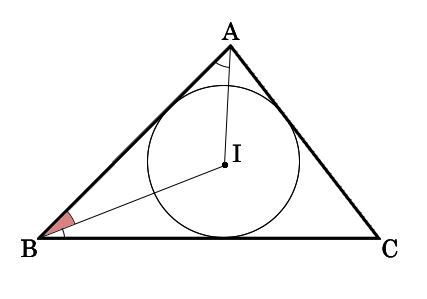Geometry

# Triangles - Incenter

The incenter of a triangle is the intersection of which 3 lines in the triangle?

Which of the following statements is true about the incenter?

Which of the following triangles doesn't have the incenter?

How can we find the point equidistant from all sides in a triangle?Point $I$ in the diagram above is the incenter of $\triangle{ABC}$. If $\angle{IAB}=40^\circ$ and $\angle{IBC}=30^\circ$, what is the measure of $\angle{IBA}$?

×